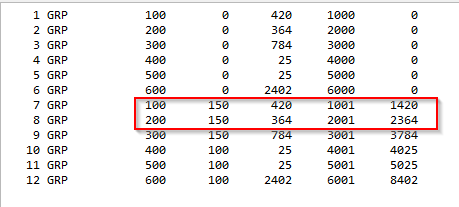# How to get nodes of a particular group from CDB with python interface?

Hello everyone,

I need to filter a few nodes (roof elements) from a structure. I filtered them using SSD and found that all the roof elements are in ´group 100 and 200´. Therefore, I attempted to retrieve all those nodes from the CDB.

To achieve this,

• I first obtained the group numbers and their corresponding maximum and minimum values from the CDB.

• Then I tried to filter just these nodes out of all the nodes based on this available Max, Min information.

Unfortunately, there is a discrepency with the nodes.
As you can see in the images below, Group 100 has a range from 1001 to a maximum of 1420, and Group 200 has from 2001 to 2364. However, from the SSD, nodes of Group 200 have numbers in other series (eg:1559, 1573), instead of starting from 2001.

Could someone assist me in extracting nodes based on groups from the CDB? Alternatively, if you could guide me in directly obtaining these roof elements (trusses), I would greatly appreciate it. Once I have access to the roof elements, my next step involves applying wind loads to these nodes.``````ie = c_int(0)
groups = {'Grp':[], 'Max':[], 'Min':[]}
while ie.value < 2:
datalen.value = sizeof(CGRP)
RecLen = c_int(sizeof(cgrp))
ie.value = py_sof_cdb_get(Index, 11, 0, byref(cgrp), byref(RecLen), 1)
if cgrp.m_typ != 0: # This makes sure you only store the groups with beam elements
groups['Grp'].append(cgrp.m_ng)
groups['Max'].append(cgrp.m_max)
groups['Min'].append(cgrp.m_min)

# Always read the length of record before sof_cdb_get is called
RecLen = c_int(sizeof(cgrp))
df_groups = pd.DataFrame.from_dict(groups)
# +============================================================================+ Getting crossection details of nodes
ie = c_int(0)
nodes = {'Node':[], 'Grp':[], 'X':[], 'Y':[], 'Z':[]}
while ie.value < 2:
datalen.value = sizeof(CNODE)
RecLen = c_int(sizeof(cnode))
ie.value = py_sof_cdb_get(Index, 20, 00, byref(cnode), byref(RecLen), 1)
nodes['Node'].append(cnode.m_nr)  # node-number
nodes['X'].append(cnode.m_xyz) # x coordinates
nodes['Y'].append(cnode.m_xyz) # y coordinates
nodes['Z'].append(cnode.m_xyz)  # z coordinates
nodes['Grp'].append(0)

# Always read the length of record before sof_cdb_get is called
RecLen = c_int(sizeof(cnode))

for i, node in enumerate(nodes['Node']):
for index, row in df_groups.iterrows():
grp = row['Grp']
Min = row['Min']
Max = row['Max']
if Min - 1 <= node <= Max + 1:
nodes['Grp'][i] = grp
break
``````

In the cdbase help manual, below is stated. Could that be it?

Nodes may have any external number, but are in an internal
sequence defining an optimum bandwidth. The internal number is
used only in this record. All element nodes or other references
are on global numbers. So you have to use one or two arrays
defining the mapping of internal to external nodes:

``````   INR(MKNOT)  defines the internal number of a node II=INR(NR)
INRE(NKNOT) defines the reverse mapping, i.e NR=INRE(II)

Examples: XYZ(IK(i),1:3)  are the coordinates for node i

DO N=1,MKNOT
IF(INR(N).NE.0) THEN
perform operations on node n in sorted order
ENDIF
ENDDO

Each node derived from a geometric point 32/NR has the external
number NR.
For SOFIMESH meshes all nodes being part of the structural mesh
have the NCOD&2 Bit. For result meshes for p-elements the internal
nodes do not have this bit and NN is equal the element number
they are derived from.
``````

Groups are for elements only.
A spring, beam or shell element is part of a group.

A node on the other hand is just a point of an FE mesh connecting elements, but isn’t part of a group itself

In your case the available elements in groups are beams (100) and trusses (150). 0 is the basic data for the group.

If you want apply loads using groups you either:

• Apply them on the truss/beam elements
• Get the nodes that the truss/beam elements are attached to first and then apply them to the nodes.
1 Like

Now It makes sense why the truss element number and group number matches. Thanks @sfr